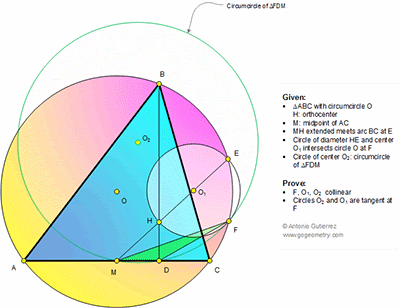## Wednesday, July 15, 2015

### Geometry Problem 1137: Triangle, Circumcircle, Orthocenter, Midpoint, Arc, Collinear Points, Tangent Circles

Level: Mathematics Education, High School, Honors Geometry, College.

Click the diagram below to enlarge it.#### 1 comment:

1.http://s14.postimg.org/j7s4od1u9/pro_1137.png

Draw points P, Q, S per attached sketch
Observe that H, M, P are collinear and AC is the perpendicular bisector of HQ. ( see other problem)
With manipulation of angles we will get ∠ (EHF)= ∠ (BPF)= ∠ (HQS)= ∠ (QHS)= ∠ (SMH) …( see sketch)
We will get the following results:
HF//AC and MH tangent to circumcircle of triangle QHF
S is the circumcenter of triangle QHF
In right triangle MHS with altitude HD we have SH^2=SF^2=SD.SM
So SF tangent to circuncircle of MDF
SH ⊥ HE and SH=SF => SF tangent to circumcircle of HFE
So SF is tangent to both circles O1 and O2